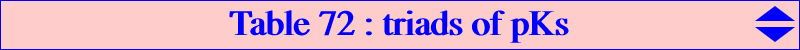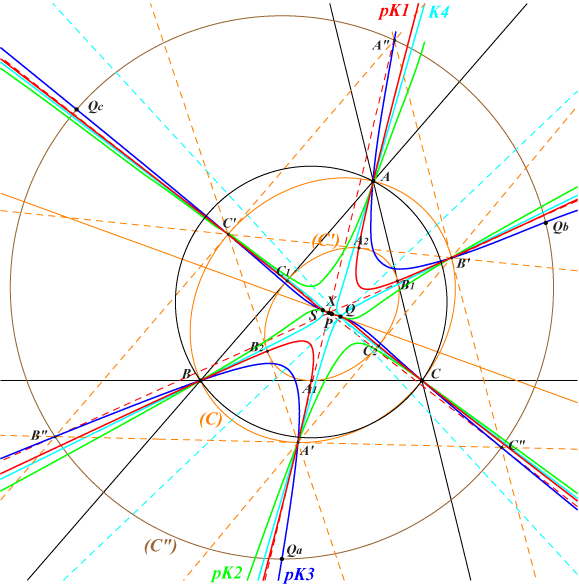Consider a pivotal cubic pK1 with pole Ω = p : q : r, pivot P = u : v : w, isopivot Q = Ω ÷ P, tertiary pivot S = P-Ceva Q. Recall that the tangentials of P and Q are Q and S rrespectively. The tangent at P to pK1 is the line PQ and the tangent at Q is the line (L) = QS. The polar conic (C) of Q is the circum-conic with perspector Q. pK1 and (L)⋃(C) generate a pencil of circum-cubics which contains : • pK2 = pK(Q x S, Q) passing through the vertices A1, B1, C1 of the cevian triangle of Q. These points obviously lie on (C'), the inconic with perspector Q. • pK3 = pK(Q^2, S) passing through the vertices Qa, Qb, Qc of the anticevian triangle of Q. Note that pK3 is anharmonically equivalent to pK1. • a nodal cubic K4 with node Q. The nine base points of the pencil are A, B, C, Q counted twice, S, and three other points A', B', C' where the tangents to pK1 concur at X. The conic (C') is inscribed in triangle A'B'C' and its points of tangency A2, B2, C2 lie on pK1. The tangents at A', B', C' to (C) meet at A", B", C" which lie on pK3, on the tangents at A', B', C' to pK1 and on a circum-conic (C") of the anticevian triangle of Q. (C") is the locus of poles in (C) of the tangents to (C'). Its perspector is Q^2 ÷ S and its equation is : (∑ p v^2 w^2) (∑ p v w y z) + (uvw)^2 (∑ q r x^2) = 0. A neat example is given at K317 where the associated pKs are K002 and K101. *** In the figure below, Ω = Q = X(1), P = X(2), S = X(9) hence pK1 = K363 = pK(X1, X2), pK2 = pK(X55, X1), pK3 = K351 = pK(X6, X9). (C) is the circum-conic with perspector X(1) and center X(9), (C') is the Steiner inellipse, (C") is the circle with center X(40) and radius 2R. X = a (a^2+2 a b-3 b^2+2 a c-26 b c-3 c^2) : : , SEARCH = 2.92619696920451.